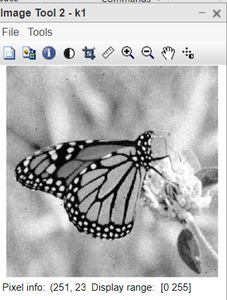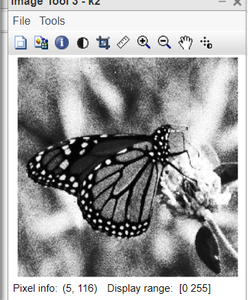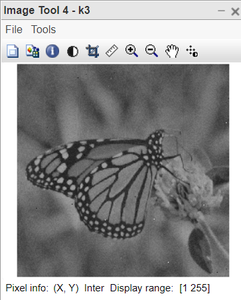Open In App

# How spatial resolution of a digitized image is different from brightness resolution in MATLAB?

A digital image is represented in the form of a 2D matrix of integers/floats, in which each element of the matrix represents pixel intensity. This intensity distribution of images depicted in the form of 2D matrices is called Spatial Domain. The intensity value depends on the bit-depth of the image. For example, if bit-depth is 8bit then pixel intensity can take values from 0 to 255.

## Why spatial resolution is important?

Spatial resolution is important as it influences how sharply we see the objects. The key parameter is not just the number of pixels in each row or column of the display, but the angle subtended, by each of these pixels on the viewer’s retina.

## What is spatial resolution?

Since we know by now that each digital image is just the collection of pixels in 2D matrix format. Now we define spatial resolution as a term that refers to the number of pixels that are used to construct a digital image. When we say that a particular digital image is having higher spatial resolution than another image, it simply means that the higher spatial resolution image is composed of more pixels than the lower spatial resolution image for the same dimensions of the imaging part. It is important to note that we are talking about the same dimensions of both images.

Spatial resolution can determine the quality of an image and describe how detailed an object can be represented by the image. It has importance in medical images such as ultrasound tomography and CT scan.

## Brightness Resolution

We know that a digital image is a collection of pixels, arranged in 2D matrix format. The values held by these pixels are the intensity values. The intensity values are either integers or float numbers. These numbers represent the brightness on a relative scale. Thus we define the brightness (or luminous brightness) of a digital image as the measure of relative intensity values of the image pixels across the pixel array.

If we increase the brightness of an image, then the brightness of each pixel increase by the same amount. Similarly, when brightness is decreased, the brightness of each pixel is decreased by the same amount. Brightness is different from contrast.

The brightness of the image does not define the quality of the image.

Syntax:

dark_image = org_image – 50; // To decrease the brightness

bright_image = org_image + 50; // To increase the brightness

imtool(image_variable, [ ]); // To display the image using imtool( ) inbuilt function

Example:

## Matlab

 `% MATLAB code for reading the image``% and convert into dark and bright images.``org_image=imread(``"GFGlogo.png"``);``dark=uint8(org_image-50);``bright=uint8(org_image+50);` `% Bring the all three images to see``% the difference in brightness.``imtool(org_image, []);``imtool(dark, []);``imtool(bright, []);`

Output: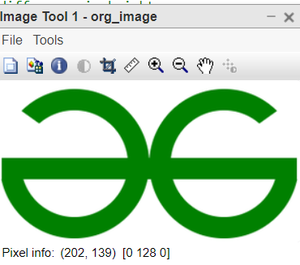Figure: Original image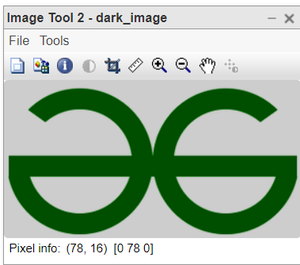Figure: Dark image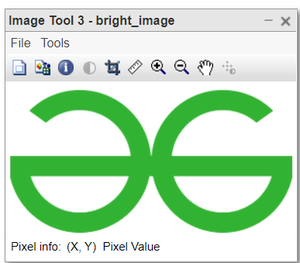Figure: Bright image

## What is the contrast of an image?

The amount of color or grayscale differentiation that exists between pixels of an image. Images having a higher contrast level generally display a greater degree of color or grayscale variation than those of lower contrast. When we increase the contrast of an image, then dark pixels get darker and light pixels get lighter.

Contrast refers to how clearly we can differentiate between two adjacent parts in an image. The contrast of an image can be identified from the histogram of the image. If the dynamic range of the histogram is wide then the corresponding image has high contrast. If the dynamic range is small then the image is said to be of low contrast. In low contrast most of the pixels of the image have the same colour therefore, it becomes difficult to distinguish the various portions of the image.

When we increase the contrast of an image then the brightness also increases, because we are widening the dynamic range of the image. That means many pixels will get light colour thus increases the brightness.

Syntax:

k=rgb2gray(k); //To convert to grayscale

k2 = histeq(k); //To enhance contrast using histogram equalisation

imtool(k,[]);  imtool(ki,[]);  where i = 1,2,3 //To display original image along with enhanced image

Example:

## Matlab

 `% MATLAB code for Contrast enhancement``% using 3 inbuilt matlab functions.``% read the colored image.``k=imread(``"poor_contrast_butterfly.jpg"``);` `%convert to grayscale.``k=rgb2gray(k);` `%contrast enhancement using adjust method.``k1 = imadjust(k);` `%contrast enhancement using histogram equalisation.``k2 = histeq(k);` `%contrast enhancement using adaptive histogram equalisation.``k3 = adapthisteq(k);` `%display original image along with enhanced image.``imtool(k,[]);``imtool(k1,[]);``imtool(k2,[]);``imtool(k3,[]);`

Output:Figure: Original image